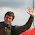## Saturday, 15 March 2014

### Plotting the Mandelbrot Set on the ZX SpectrumThe Mandelbrot set is a fractal which iterates the equation zn+1 = zn² + c in the complex plane and plots which points tend to infinity. Plotting the set with Sinclair BASIC takes over 24 hours so I was curious how much faster it would be in assembly.

It turns out if we use fast 16-bit fixed-point arithmetic we can plot the Mandelbrot in about 5 minutes. To minimise multiplications each iteration is calculated as:

rn+1 = ( rn + in ) × ( rn - in ) + x

in+1 = 2 × in × rn + y

The following test is used to detect points which tend to infinity:

|in| + |rn| ≥ 2 × √ 2.
```  org 60000
ld de,255*256+191
XLOOP:
push de
ld hl,-180   ; x-coordinate
ld e,d
call SCALE
ld (XPOS),bc
pop de
YLOOP:
push de
ld hl,-96    ; y-coordinate
call SCALE
ld (YPOS),bc
ld hl,0
ld (IMAG),hl
ld (REAL),hl
ld b,15      ; iterations
ITER:
push bc
ld bc,(IMAG)
ld hl,(REAL)
or a
sbc hl,bc
ld d,h
ld e,l
call FIXMUL
ld de,(XPOS)
ld de,(REAL)
ld (REAL),hl
ld hl,(IMAG)
call FIXMUL
rla
ld de,(YPOS)
ld (IMAG),hl
call ABSVAL
ex de,hl
ld hl,(REAL)
call ABSVAL
ld a,h
cp 46        ; 46 ≅ 2 × √ 2 << 4
pop bc
jr nc,ESCAPE
djnz ITER
pop de
call PLOT
db 254       ; trick to skip next instruction
ESCAPE:
pop de
dec e
jr nz,YLOOP
dec d
jr nz,XLOOP
ret

FIXMUL:        ; hl = hl × de >> 24
call MULT16BY16
ld a,b
ld b,4
FMSHIFT:
rla
djnz FMSHIFT
ret

SCALE:         ; bc = (hl + e) × zoom
ld d,0
ld de,48     ; zoom

MULT16BY16:    ; hl:bc (signed 32 bit) = hl × de
xor a
call ABSVAL
ex de,hl
call ABSVAL
push af
ld c,h
ld a,l
call MULT8BY16
ld b,a
ld a,c
ld c,h
push bc
ld c,l
call MULT8BY16
pop de
ld b,l
ld l,h
ld h,a
pop af
rra
ret nc
ex de,hl
xor a
ld h,a
ld l,a
sbc hl,bc
ld b,h
ld c,l
ld h,a
ld l,a
sbc hl,de
ret

MULT8BY16:     ; returns a:hl (24 bit) = a × de
ld hl,0
ld b,8
M816LOOP:
rla
jr nc,M816SKIP
M816SKIP:
djnz M816LOOP
ret

PLOT:          ; plot d = x-axis, e = y-axis
ld a,7
and d
ld b,a
inc b
ld a,e
rra
scf
rra
or a
rra
ld l,a
xor e
and 248
xor e
ld h,a
ld a,d
xor l
and 7
xor d
rrca
rrca
rrca
ld l,a
ld a,1
PLOTBIT:
rrca
djnz PLOTBIT
or (hl)
ld (hl),a
ret

ABSVAL:        ; returns hl = |hl| and increments
bit 7,h      ; a if the sign bit changed
ret z
ld b,h
ld c,l
ld hl,0
or a
sbc hl,bc
inc a
ret

XPOS:dw 0
YPOS:dw 0
REAL:dw 0
IMAG:dw 0
```

1.It looks as though you've adapted Gauss's complex multiplication algorithm (see https://en.wikipedia.org/wiki/Multiplication_algorithm#Gauss.27s_complex_multiplication_algorithm) to your need for squaring followed by adding, then streamlined it. But that wikipedia article states "This algorithm uses only three multiplications, rather than four, and five additions or subtractions rather than two. If a multiply is more expensive than three adds or subtracts, as when calculating by hand, then there is a gain in speed. On modern computers a multiply and an add can take about the same time so there may be no speed gain." If there is a gain, you could gain even more by replacing that multiplication by 2 with a doubling by addition (I haven't looked at your code, maybe you already did).

It also looks as though your test for unbounded divergence is based on the result that "... if the absolute value of P_c^n(0) ever becomes larger than 2, the sequence will escape to infinity" (see https://en.wikipedia.org/wiki/Mandelbrot_set#Basic_properties), with you simply testing for your simpler norm at a value that always lies outside the cartesian norm of 2 (since anything passing your test has met the requirements of the result, and anything that diverges unboundedly must necessarily get past your norm's boundary at some point). You could possibly simplify your test further by substituting a slightly larger but rational (better still, dyadic rational, i.e. with finitely many non-zero binary places) test criterion not involving the square root of 2 (again, maybe you already did).

2.You can speed it up considerably by not using multiplications at all, since you're just suqaring anyway. Squares can be determined from a lookup table, which, in case of the kind of 16-bit arithmetics that you use takes up 32 kilobytes and can be generated in a blink of an eye.

Thus:
rₙ₊₁ = x + (rₙ)² - (iₙ)²
iₙ₊₁ = y + (rₙ + iₙ)² - [(rₙ)² + (iₙ)²]

That makes a total of 3 lookups. Moreover, if you calculate the Euclidean norm [(rₙ)² + (iₙ)²] first, it can be used to abort the iteration if it grows beyond 4.